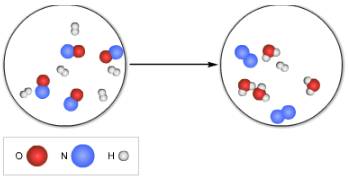# Problem: For the reaction shown below, what is the chemical formula for the limiting reactant?Write the balanced chemical equation for the reaction, using lowest whole-number coefficients.

###### FREE Expert Solution

We’re being asked to write the balanced chemical equation and determine the chemical formula for the limiting reagent.

For this problem, we need to do the following steps:

Step 1: Determine the excess and limiting reagent.
Step 2: Write and balance a chemical equation for the given reaction.

Step 1: Determine the excess and limiting reagent.
From the given molecular model given:

95% (293 ratings)###### Problem Details

For the reaction shown below, what is the chemical formula for the limiting reactant?

Write the balanced chemical equation for the reaction, using lowest whole-number coefficients.